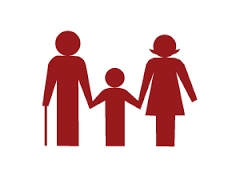# Together 23211

Lenka is three times younger than her brother Lukas; they are 20 years old. How old is Lenka, and how many are Lukas?

a =  5
b =  15

### Step-by-step explanation:

a=b/3
a+b = 20

3a-b = 0
a+b = 20

Row 2 - 1/3 · Row 1 → Row 2
3a-b = 0
1.33b = 20

b = 20/1.33333333 = 15
a = 0+b/3 = 0+15/3 = 5

a = 5
b = 15

Our linear equations calculator calculates it.Did you find an error or inaccuracy? Feel free to write us. Thank you!

Tips for related online calculators
Do you have a linear equation or system of equations and looking for its solution? Or do you have a quadratic equation?
Do you want to convert time units like minutes to seconds?